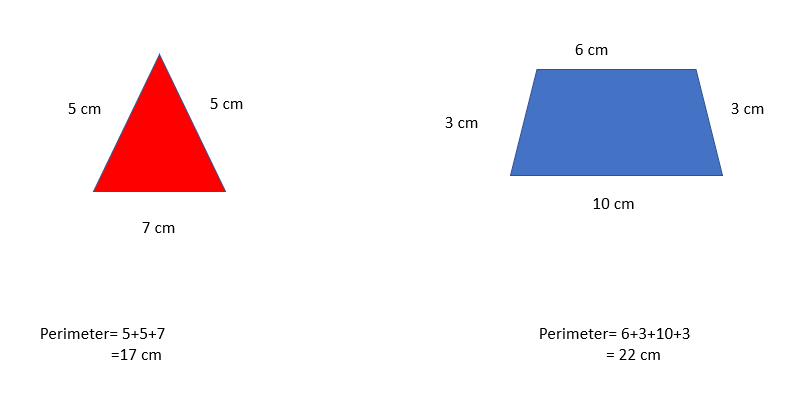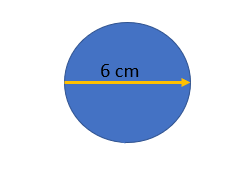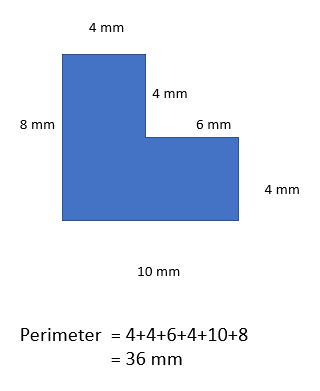# Calculating Length

## Perimeter of 2D shapes

To find the perimeter of 2D shapes simply add up the length of each sides!

Remember regular polygons have sides with the same lengths:## Circumference of circles

To find the circumference of a circle use the formula circumference = diameter x π. Eg.:Circumference = 6 x π = 18.85 (to 2 dp)

## Perimeter of compound shapes

Compound shapes are shapes that are made up of more than one. For example:For example this shape can be divided into two rectangle.

A regular pentagon has a length of 4 cm, what is the perimeter of the pentagon?
Your answer should include: 20cm / 20
A regular polygon has a perimeter of 50 mm, each side is 8 ⅓mm what is the name of the shape?
Hexagon